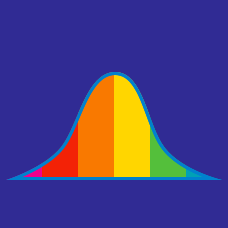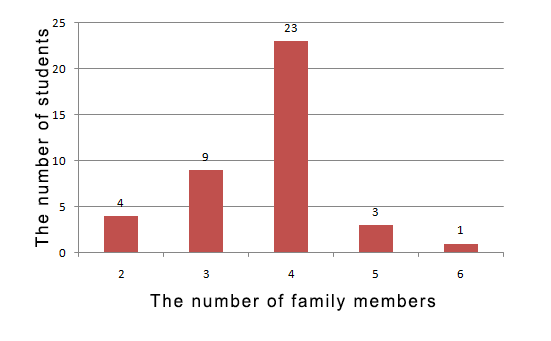Probability

# Data - Mean

What is the mean of the following three numbers:

$1, 9, 14?$

 Details and Assumptions:

• The mean of a set of numbers is the average of the set.

$\{ 5, 8, 10, 15, 27 \}$

What is the mean of the above set?

 Species Number of Legs Human 2 Dog 4 Cat 4 Ant 6 Octopus 8 Sea pig 12

Using the table above, determine the mean number of legs of the group.A group of students were asked how many family members they each have in their family, and the above graph is the frequency distribution from the survey. Determine the mean number of their family members.

$\{ 4, 8, 30 \}$

What is the mean of the above set?

×